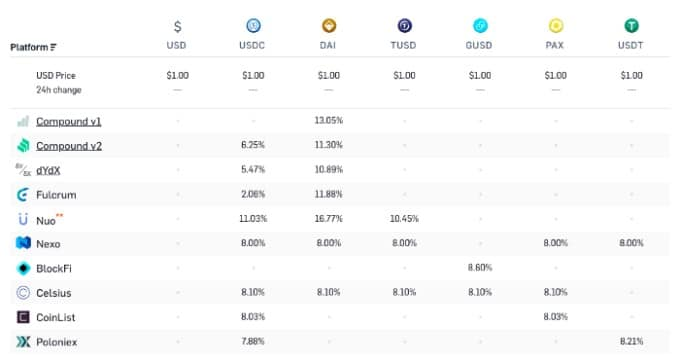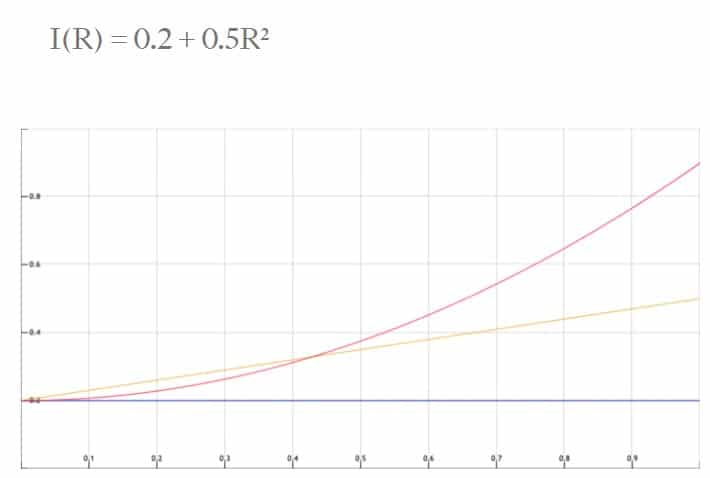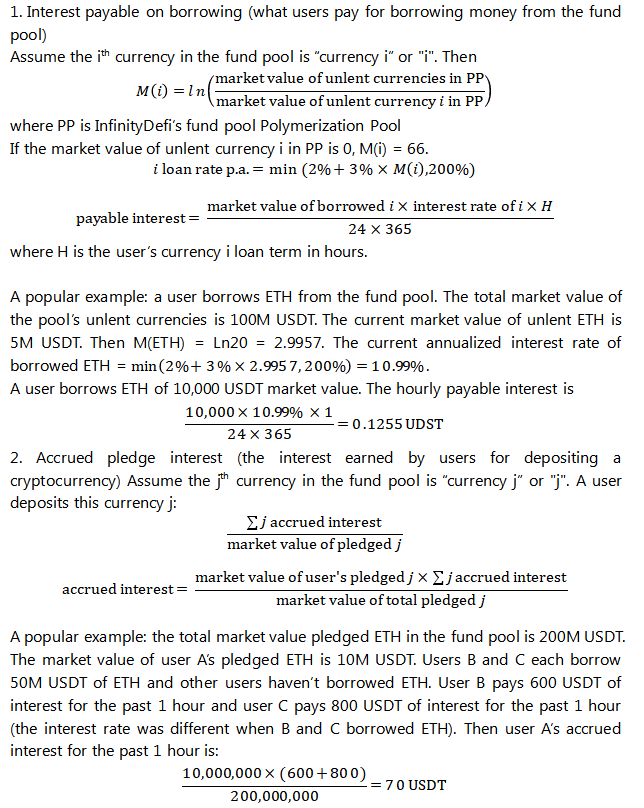# Depth Dive into How InfinityDefi Polymerization Pool Works

Place/Date: - October 22nd, 2020 at 8:46 am UTC · 5 min read
Source: InfinityDefi

Ever since its launch, InfinityDefi has been gaining extreme traction and has entered the industry mainstream. What is behind InfinityDefi’s collateral lending business model? Let’s start with the interest rate model of the youngest DeFi unicorn.

## How Old Decentralized Lending Pools Set the Interest Rate

When you open a DeFi loan aggregator, you find many assets across multiple platforms. The interest rate models for deposits and lending varies across platforms:After all, collateral lending revolves around the deposit and borrowing interest rate. A good interest rate model means a project is competitive. So how are the interest rates set?

Normally, ETH DeFi interest rate models base on supply and demand. In most cases, the interest rate of a decentralized lending pool is determined by a function that takes the pool state as input:

I(R)

where I is the interest rate and R is the utilization rate.

R = 0 means no assets are lent. R = 1 means all assets are lent.

Let’s first consider a simple case, ignore the state of the pool and use a constant interest rate:

I(R)=0.2

Lending pools with a constant interest rate set the rate at 20% in all cases. A real-world example is Huobi with its 0.1% daily. As a centralized exchange, Huobi can effectively issue an unlimited number of loans.

As for decentralized P2P platforms where loans are backed by real on-chain assets, it is usually desirable to choose an interest rate function to encourage balanced utilization. Low utilization rate is not good because the generated interest is too low. Too high utilization rate is not good because the fund pool can no longer meet the borrowing needs.

Let’s turn to a function that changes along with utilization:

I(R)=0.2+0.3R

The addition is quite meaningful. If few people borrow money, the interest rate will be lower. If many people borrow, the interest rate will be higher. Compound is an example of a lending pool with a linear model.

Next, let’s consider higher-order functions:Red line is a non-linear interest rate

Why non-linear? One reason is the ability to fine-tune various parts of the curve. In the median situation, users like a relatively stable and robust curve. In extreme cases when the fund pool almost lacks liquidity, interest rates will change quickly in an attempt to restore the balance.

Linear curves are already rewarding borrowers because they provide a rare asset when needed. The nonlinear curve does not stop there. One reason for extra rewards is the liquidity risk: if the utilization rate is high and many lenders withdraw at the same time, some of them must wait until the outstanding loans are paid off. The decentralized margin exchange DDEX and trading platform dYdX use non-linear interest rate functions.

Below are the actual interest rate functions of Compound, dYdX, and DDEX’s DAI stablecoin for reference:

Compound: 0.05 + 0.15R

dYdX: 0.15R +0.85R6

DDEX: 0.10 +0.4R4+0.5R8

## Enter Extreme Conditions!

R = 0: initial state, the lowest interest rate

An interest point to consider is R = 0. This is the extreme case when 100% of the assets are available and platform has the lowest interest rate. Let’s enter the value:

Compound (0) = 0.05

dYdX (0) = 0

DDEX (0) = 0.10

The idea of non-zero interest rates is that stablecoins should require a risk-free interest rate equal to or higher than US dollar.

R = 1: overused pool, the highest interest rate

The other extreme. All assets in the pool are lent and the pool is illiquid. The platform has the highest interest rate:

Compound (1) = 0.2

dYdX (1) = 1

DDEX (1) = 1

The highest interest rate on Compound is 50%. DDEX and dYdX have 100%. The dYdX and DDEX pools are mainly used to support margin trading. The lent lending pool is in a bad position because trading is no longer possible. Compound has a more general lending pool with an IOU system (cToken) therefore, high utilization is okay.

Different curves are useful for different pools. After all, a good interest rate model can help approximate equilibrium interest rates when the total lending demand equals the total lending supply. Algorithmic interest rate models are the most popular solutions today because they work well and are simple and easy for on-chain implementation.

## What’s New about INFI’s Algorithmic Interest Rate Model?

INFI resembles Compound, except that its arbitrage space and lending are more flexible than traditional collateral projects. Besides the functions traditional DeFi has, INFI has an adjustment mechanism in its Polymerization Pool to change interest rates dynamically according to the ratio of coins. Thus, there is balance between supply and demand of any coin.

INFI’s algorithmic interest rate model suits the investment preferences of cryptocurrency users better. Below is the algorithmic interest rate model of InfinityDefi deposit and lending.As per the above formula, the tighter the supply and demand, the higher the interest rate of a currency and the arbitrage chances. Floating interest rates can help arbitrage and frequent arbitrage will balance the supply and demand of cryptocurrencies and eventually smooth out interest rate fluctuations.

InfinityDefi also stands out among the existing DeFi products due to its new features like secondary pledging and MVA loans. With secondary pledging, users can pledge their external collateral to other users of the platform for a better arbitrage space. MVA are multi-value-added loans where users can pledge the value-added part of collateral.

## For Those Who Scrolled Straight to the Bottom

InfinityDefi has on average 5% lower loan rate, 20% higher loan limit, and faster capital turnover.

Users get bonus equity tokens PPT for every primary and secondary pledging and loan.

The generation and distribution of PPT are decentralized. The earned PPT depends on the size and term of pledging or loan.

Users can exchange PPT to ecosystem tokens INFI instantly. INFI is the governance token and the tool for profit dividends that will be listed on exchanges.

InfinityDefi White Listing has Started:

Share: